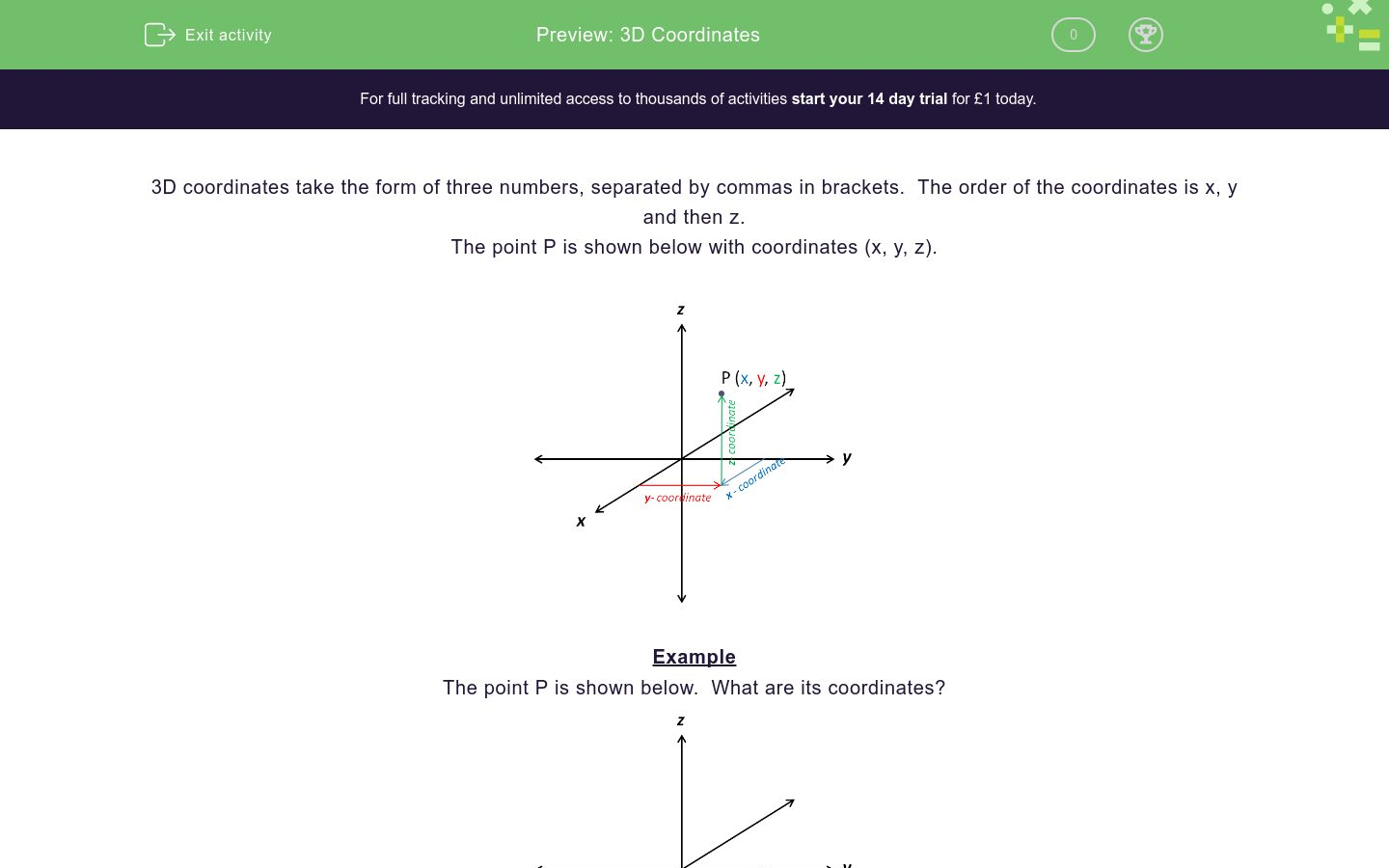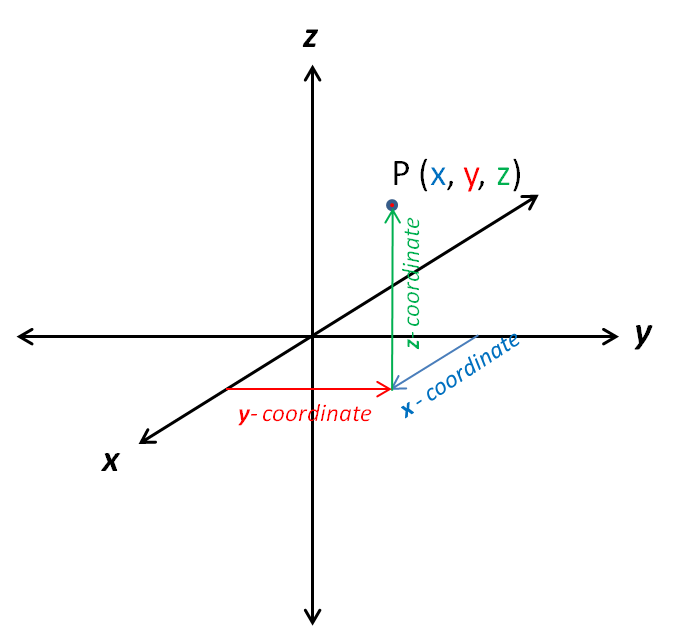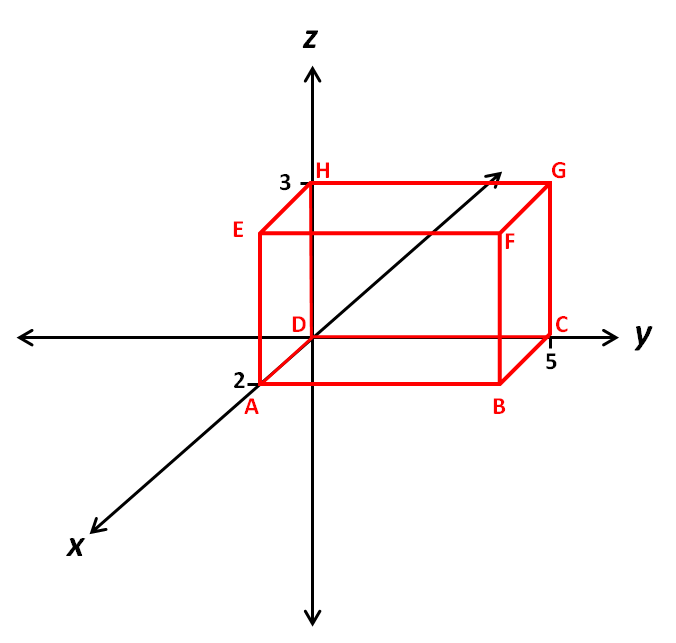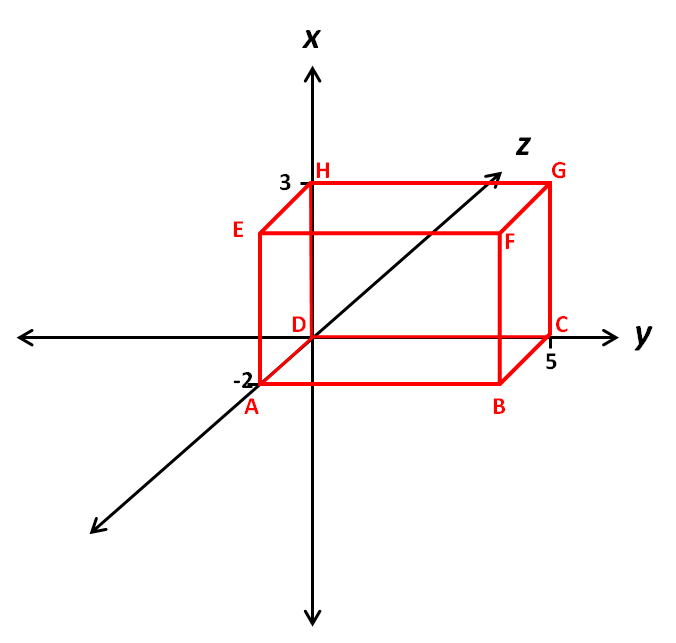# 3D Coordinates

In this worksheet, students read 3D coordinates from representations of 3D axes.Key stage:  KS 4

Curriculum topic:  Geometry and Measures

Curriculum subtopic:  Construct and Interpret Plans and Elevations of 3D Shapes

Difficulty level:### QUESTION 1 of 10

3D coordinates take the form of three numbers, separated by commas in brackets.  The order of the coordinates is x, y and then z.

The point P is shown below with coordinates (x, y, z).Example

The point P is shown below.  What are its coordinates?P is below the xy-plane so the z-coordinate is negative.

P is at (2, 3, -5).

ABCDEFGH is a cuboid.

Select the coordinates of A.(2, 5, 0)

(2, 0, 0)

(0, 2, 0)

ABCDEFGH is a cuboid.

Select the coordinates of B.(2, 5, 0)

(2, 0, 0)

(0, 5, 0)

ABCDEFGH is a cuboid.

Select the coordinates of C.(2, 5, 5)

(5, 0, 0)

(0, 5, 0)

ABCDEFGH is a cuboid.

Select the coordinates of E.(2, 0, 3)

(0, 0, 3)

(0, 3, 0)

ABCDEFGH is a cuboid.

Select the coordinates of F.(2, 0, 3)

(2, 5, 3)

(2, 3, 5)

ABCDEFGH is a cuboid.

Select the coordinates of C.(0, 5, 0)

(0, 0, 5)

(5, 0, 0)

ABCDEFGH is a cuboid.

Select the coordinates of E.(0, 3, 2)

(0, 2, 3)

(2, 0, 0)

ABCDEFGH is a cuboid.

Select the coordinates of E.(0, 3, 2)

(0, -2, 3)

(3, 0, -2)

ABCDEFGH is a cuboid.

Select the coordinates of B.(0, 5, 2)

(0, 5, -2)

(-2, 5, 0)

ABCDEFGH is a cuboid.

Select the coordinates of F.(3, 5, 2)

(3, 5, -2)

(-2, 5, 3)

• Question 1

ABCDEFGH is a cuboid.

Select the coordinates of A.(2, 0, 0)
EDDIE SAYS
2 along the x-axis
0 along the y-axis
0 along the z-axis
• Question 2

ABCDEFGH is a cuboid.

Select the coordinates of B.(2, 5, 0)
EDDIE SAYS
2 along the x-axis
5 along the y-axis
0 along the z-axis
• Question 3

ABCDEFGH is a cuboid.

Select the coordinates of C.(0, 5, 0)
EDDIE SAYS
0 along the x-axis
5 along the y-axis
0 along the z-axis
• Question 4

ABCDEFGH is a cuboid.

Select the coordinates of E.(0, 3, 0)
EDDIE SAYS
2 along the x-axis
0 along the y-axis
3 along the z-axis
• Question 5

ABCDEFGH is a cuboid.

Select the coordinates of F.(2, 5, 3)
EDDIE SAYS
2 along the x-axis
5 along the y-axis
3 along the z-axis
• Question 6

ABCDEFGH is a cuboid.

Select the coordinates of C.(5, 0, 0)
EDDIE SAYS
5 along the x-axis
0 along the y-axis
0 along the z-axis
• Question 7

ABCDEFGH is a cuboid.

Select the coordinates of E.(0, 3, 2)
EDDIE SAYS
0 along the x-axis
3 along the y-axis
2 along the z-axis
• Question 8

ABCDEFGH is a cuboid.

Select the coordinates of E.(3, 0, -2)
EDDIE SAYS
3 along the x-axis
0 along the y-axis
-2 along the z-axis
• Question 9

ABCDEFGH is a cuboid.

Select the coordinates of B.(0, 5, -2)
EDDIE SAYS
0 along the x-axis
5 along the y-axis
-2 along the z-axis
• Question 10

ABCDEFGH is a cuboid.

Select the coordinates of F.(3, 5, -2)
EDDIE SAYS
3 along the x-axis
5 along the y-axis
-2 along the z-axis
---- OR ----

Sign up for a £1 trial so you can track and measure your child's progress on this activity.

### What is EdPlace?

We're your National Curriculum aligned online education content provider helping each child succeed in English, maths and science from year 1 to GCSE. With an EdPlace account you’ll be able to track and measure progress, helping each child achieve their best. We build confidence and attainment by personalising each child’s learning at a level that suits them.